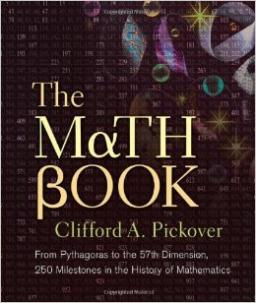# Earlier 4970

Tomáš and Lukáš read the same book. Tomas read 21 pages a day and read it one day earlier than Luke, who read 18 pages a day. How many pages did the book have?

s =  126

### Step-by-step explanation:

s=21t
s = 18(t+1)

s=21·t
s = 18·(t+1)

s-21t = 0
s-18t = 18

Row 2 - Row 1 → Row 2
s-21t = 0
3t = 18

t = 18/3 = 6
s = 0+21t = 0+21 · 6 = 126

s = 126
t = 6

Our linear equations calculator calculates it.Did you find an error or inaccuracy? Feel free to write us. Thank you!

Tips for related online calculators
Do you have a linear equation or system of equations and looking for its solution? Or do you have a quadratic equation?